Courses

# Test: Sequences And Series (CBSE Level) - 1

## 25 Questions MCQ Test Mathematics (Maths) Class 11 | Test: Sequences And Series (CBSE Level) - 1

Description
This mock test of Test: Sequences And Series (CBSE Level) - 1 for JEE helps you for every JEE entrance exam. This contains 25 Multiple Choice Questions for JEE Test: Sequences And Series (CBSE Level) - 1 (mcq) to study with solutions a complete question bank. The solved questions answers in this Test: Sequences And Series (CBSE Level) - 1 quiz give you a good mix of easy questions and tough questions. JEE students definitely take this Test: Sequences And Series (CBSE Level) - 1 exercise for a better result in the exam. You can find other Test: Sequences And Series (CBSE Level) - 1 extra questions, long questions & short questions for JEE on EduRev as well by searching above.
QUESTION: 1

### The next term of the sequence 1, 2, 4, 7,11,…. is

Solution:

The given series is: 1,2,4,7,11,...
Difference between second and first term = 2 - 1 = 1
Difference between third and second term = 4 - 2 = 2
Difference between fourth and third term = 7 - 4 = 3
Difference between fifth and fourth term = 11 - 7 = 4
Difference between sixth and fifth term = 16 - 11 = 5

QUESTION: 2

### The next term of the sequence, 2, 6, 12, 20, …..is

Solution:

The given sequence is based on the following pattern: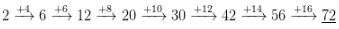∴ Required number = 30.

QUESTION: 3

### The next of the series 3/2 + 5/4 + 9/8 + 17/16 .....is

Solution: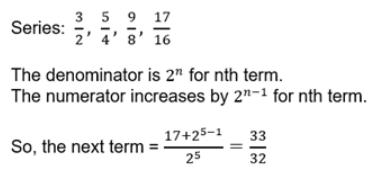QUESTION: 4

Which term of the sequence 8 – 6i, 7 – 4i, 6 – 2i, ….is a real number ?

Solution:

a = 8−6i
d = 7−4i−8+6i
= −1+2i
an = a+(n−1)d
a+ib = 8−6i+(n−1)(−1+2i)
a+ib = 8−6i+(−1)(n−1)+(n−1)2i
= − 6+2(n−1)=0
= 2(n−1) = 6
n = 4
an = 8−6i+(4−1)(−1+2i)
= 8−6i−3+6i = 5
4th term = 5

QUESTION: 5

All the terms in A.P., whose first term is a and common difference d are squared. A different series is thus formed. This series is a

Solution: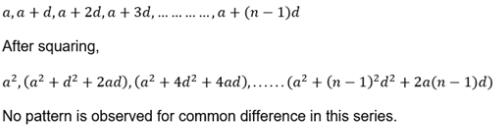QUESTION: 6

If a, 4, b are in A.P.; a, 2, b are in G.P.; then a, 1, b are in

Solution:
QUESTION: 7

The eleventh term of the sequence 1, 1, 2, 3, 5, 8, 13, 21, 34, ….. is

Solution:

The sequence is the Fibonacci series
1+1 = 0
1+2 = 3
2+3 = 5
3+5 = 8
5+8 = 13
8+13 = 21
13+21 = 34
21+34 = 55
34+55 = 89
The 11th term will be 89.

QUESTION: 8

In an A.P., sum of first p terms is equal to the sum of first q terms. Sum of its first p + q terms is

Solution:

Sp = Sq
⇒  p/2(2a+(p−1)d) = q/2(2a+(q−1)d)
⇒ p(2a+(p−1)d) = q(2a+(q−1)d)
⇒ 2ap + p2d − pd = 2aq + q2d − qd
⇒ 2a(p−q) + (p+q)(p−q)d − d(p−q) = 0
⇒ (p−q)[2a + (p+q)d − d] = 0
⇒ 2a + (p+q)d − d = 0
⇒ 2a + ((p+q) − 1)d = 0
⇒ Sp+q = 0

QUESTION: 9

The sum of 40 A.M.’s between two number is 120. The sum of 50 A.M.’s between them is equal to

Solution:

Let A1, A2, A3, ........ , A40 be 40 A.M's between two numbers 'a' and 'b'.
Then,
a, A1, A2, A3, ........ , A40, b is an A.P. with common difference d  = (b - a)/(n + 1) = (b - a)/41
[ where n = 40]
now, A1, A2, A3, ........ , A40  = 40/2( A1 + A40)
A1, A2, A3, ........ , A40 = 40/2(a + b)
[ a, A1, A2, A3, ........ , A40, b is an Ap then ,a + b = A1 + A40]
sum of 40A.M = 120(given)
120= 20(a + b)
=> 6 = a + b ----------(1)
Again, consider B1, B2, ........ , B50  be 50 A.M.'s between two numbers a and b.
Then, a, B1, B2, ........ , B50, b will be in A.P. with common difference = ( b - a)/51
now , similarly,
B1, B2, ........ , B50 = 50/2(B1 + B2)
= 25(6) ----------------from(1)
= 150

QUESTION: 10

In an A.P., sum of first p terms is q and sum of first q terms is p. Sum of its p + q terms is

Solution:

Let the first term of the given AP be ‘a' and the common difference be ‘d'. Then, the sum of first ‘n' terms of the AP is given by:
Sn =  n/2 {2a+(n-1)d} …….(1)
Here, it is given that:
Sp = q and Sq = p
Using (1), we get:-
q = p/2 {2a+(p-1)d}
and p = q/2 {2a+(q-1)d}
i.e. 2a + (p-1)d = 2q/p …..(2)
and 2a + (q-1)d = 2p/q …..(3)
Subtracting (3) from (2), we get:
(p - 1 - q + 1)d = 2q/p - 2p/q
So, d = 2(q2 - p2)/pq(p-q)
i.e. d = -2(p+q)/pq
Now, substituting the value of ‘d' in eq.n (2), we get:
2a + (p-1){-2(p+q)/pq} = 2q/p
i.e. 2a= 2q/p + 2(p-1)(p+q)/pq
This gives:
a = (p2 + q2 - p - q + pq)/pq
So, we have
Sp+q = (p+q)/2 { 2(p2+q2-p-q+pq)/pq - (p+q-1) 2 (p+q)/pq}
i.e. Sp+q = (p+q)/pq { p2+q2-p-q+pq-p2-pq-qp-q2+p+q}
So, Sp+q = -(p+q)

QUESTION: 11

pth term of an A.P. is q and qth term is p, its (p+ q)th term is

Solution:
QUESTION: 12

pth term of an H.P. is qr and qth term is pr, then rth term of the H.P. is

Solution:

Given pth term of HP = qr
So pth term of AP = 1/qr
a+(p−1)d = 1/qr....(1)
and qth term of HP = pr
so qth term of AP = 1/pr
a + (q−1)d = 1/pr.....(2)
subtracting equation 1 and 2 we get,
(p−q)d = (p−q)/pqr
d = 1/pqr
Now from equation 1,
a = 1/qr − (p−1)/pqr
= (p−p+1)/pqr = 1/pqr
So rth term of AP = a+(r−1)d = 1/pqr + (r − 1)/pqr = 1/pq
So, rth term of HP = pq

QUESTION: 13

The value of b for which the roots of the equation six x = b are in A.P. is

Solution: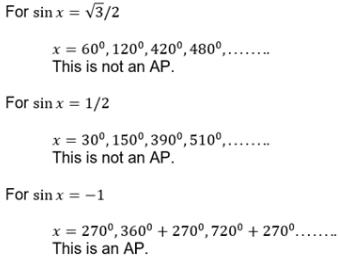QUESTION: 14

The number of numbers between n and n2 which are divisible by n is

Solution:

Between n & n2, numbers divisible by n are:
2n, 3n, 4n, ….... (n – 1)n
No. of numbers = (n – 1) – 2 + 1 = n – 2

QUESTION: 15

The number of terms common to the Arithmetic progressions 3, 7, 11, …., 407 and 2, 9, 16, …., 709 is

Solution:

First A.P′s sequence is 3,7,11,....,407
General term will be 4k+3    k≤101
Second A.P's sequence is 2,9,16,....,709
General term will be 7p+2    p≤101
The common terms will be 51,79,...,28m + 51
28m + 51 ≤ 407
⟹ 28k ≤ 356
⟹ k ≤ 12.71
And adding the 2 starting number count of the 2 A.P′s i.e. 3 & 2
Number of common terms will be 12 + 2 = 14

QUESTION: 16

If a, b, c are in A. P. as well as in G.P.; then

Solution:
QUESTION: 17

The 20th term of the series 2×4+4×6+6×8+... is

Solution: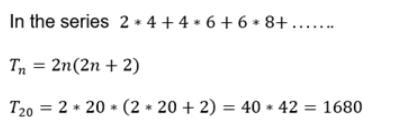QUESTION: 18

The ratio of first to the last of n A.m.’s between 5 and 25 is 1 : 4. The value of n is

Solution: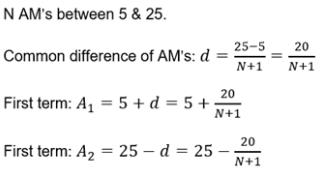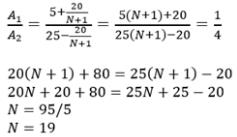QUESTION: 19

The next term of the sequence 1, 3, 6, 10, …. Is

Solution:
QUESTION: 20

If A, G, H denote respectively the A.M., G.M. and H.M. between two unequal positive quantities then

Solution:
QUESTION: 21

If A, G and H denote respectively, the A.M., G.M. and H.M. between two positive numbers a and b, then A - G is equal to

Solution:
QUESTION: 22

If a, b, c, d are in H.P., then ab + bc + cd is

Solution:

Since a,b,c are in H.P, so b = 2ac/(a+c).
Also, b,c,d are in H.P, so c = 2bd/(b+d).
Therefore, (a+c)(b+d) = 2ac/b × 2bd/c

QUESTION: 23

The sum of all 2-digited numbers which leave remainder 1 when divided by 3 is

Solution:

The 2-digit number which when divided by 3 gives remainder 1 are: 10, 13, 16, ...97
Here a = 10, d = 13 - 10 = 3
tn = 97
nth term of an AP is tn = a + (n – 1)d
97 = 10 + (n – 1)3
⇒ 97 = 10 + 3n – 3
⇒ 97 = 7 + 3n
⇒ 3n = 97 – 7 = 90
∴ n = 90/3 = 30
Recall sum of n terms of AP,
= 15[20 + 87] = 15 × 107 = 1605

QUESTION: 24

The number of numbers between 105 and 1000 which are divisible by 7 is

Solution:

100÷7= 14 2/7 , 7×15=105

1000÷7=142 6/7 , 7×142=994

15th to 142 th multiples of 7 are there in between 100 and 1000 .

No of them are142–14= 128

so 128 is the ans

QUESTION: 25

The next term of the sequence 1, 5, 14, 30, 55, …… is

Solution: